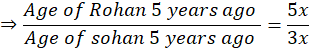Question of The Day25-11-2021

The ratio of age of Rohan and Sohna 5 years ago was 5 : 3 and the sum present age of both is 98 years. What is the age of Sohan 7 years hence?

Correct Answer : a ) 45 years

Explanation :

According to the question

Let the common multiple be x

The ratio of age of Rohan and Sohna 5 years ago was 5 : 3Present age of Rohan = 5x + 5

Present age of Sohan = 3x + 5

Sum of present age of Both = 5x + 5 + 3x + 5

8x + 10

8x + 10 = 98

8x = 98 – 10

8x = 88

x = 11

Present age of Sohan = 3x + 5

3 * 11 + 5

33 + 5

38 years

Age of sohan 7 years hence = 38 + 7

45 years

Hence, (a) is the correct answer.0